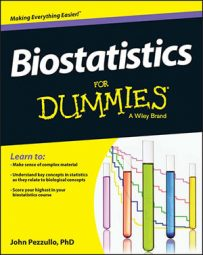##### Biostatistics For DummiesModern statistical software makes it easy for you to analyze your data in most of the situations that you’re likely to encounter (summarize and graph your data, calculate confidence intervals, run common significance tests, do regression analysis, and so on). But occasionally you may run into a problem for which no preprogrammed solution exists. Deriving new statistical techniques can involve some very complicated mathematics, and usually only a professional theoretical statistician attempts to do so.

But there’s a simple yet general and powerful way to get answers to a lot of statistical questions, even if you aren’t a math whiz. It’s called simulation, or the Monte-Carlo technique.

Statistics is the study of random fluctuations, and most statistical problems really come down to the question “What are the random fluctuations doing?” Well, it turns out that computers are very good at drawing random numbers from a variety of distributions. With the right software, you can program a computer to make random fluctuations that embody the problem you’re trying to solve; then you can simply see what those fluctuations did. You can then rerun this process many times and summarize what happened in the long run.

The simulation approach can be used to solve problems in probability theory, determine statistical significance in common or uncommon situations, calculate the power of a proposed study, and much more. Here’s a simple, if somewhat contrived, example of what simulation can do:

What’s the chance that the product of the IQs of two randomly chosen people is greater than 12,000?

IQs are normally distributed, with a mean of 100 and a standard deviation of 15. (And don’t ask why anyone would want to multiply two IQ scores together; it’s just an example!)

As simple as this question may sound, it’s a very difficult problem to solve exactly, and you’d have to be an expert mathematician to even attempt it. But it’s very easy to get an answer by simulation. Just do this:

1. Generate two random IQ numbers (normally distributed, m = 100, sd = 15).

2. Multiply the two IQ numbers together.

3. See whether the product is greater than 12,000.

4. Repeat Steps 1–3 a million times and count how many times the product exceeds 12,000.

5. Divide that count by a million, and you have your probability.

This simulation can be set up using the free Statistics 101 program or even Excel. Using R software, the five steps can be programmed in a single line:

`sum(rnorm(1000000,100,15)*rnorm(1000000,100,15)>12000)/1000000`

Even if you’re not familiar with R’s syntax, you can probably catch the drift of what this program is doing.

• Each “rnorm” function generates a million random IQ scores.

• The “*” multiplies them together pairwise.

• The “>” compares each of one million products to 12,000.

• The “sum” function adds up the number of times the comparison comes out true (true counts as 1; false counts as 0).

• The “/” divides the sum by a million.

R prints out the results of “one-liner” programs like this one without your having to explicitly tell it to. When one person ran this program on his desktop computer, it computed for about a half-second and then printed the result: 0.172046. Then he ran it again, and it printed 0.172341. That’s a characteristic of simulation methods — they give slightly different results each time you run them. And the more simulations you run, the more accurate the results will be. That’s why the preceding steps ask for a million repetitions. You won’t get an exact answer, but you’ll know that the probability is around 0.172, which is close enough.